## 1.2 Basic Classes of Functions

### Learning Objectives

• Calculate the slope of a linear function and interpret its meaning.
• Recognize the degree of a polynomial.
• Find the roots of a quadratic polynomial.
• Describe the graphs of basic odd and even polynomial functions.
• Identify a rational function.
• Describe the graphs of power and root functions.
• Explain the difference between algebraic and transcendental functions.
• Graph a piecewise-defined function.
• Sketch the graph of a function that has been shifted, stretched, or reflected from its initial graph position.

We have studied the general characteristics of functions, so now let’s examine some specific classes of functions. We begin by reviewing the basic properties of linear and quadratic functions, and then generalize to include higher-degree polynomials. By combining root functions with polynomials, we can define general algebraic functions and distinguish them from the transcendental functions we examine later in this chapter. We finish the section with examples of piecewise-defined functions and take a look at how to sketch the graph of a function that has been shifted, stretched, or reflected from its initial form.

# Linear Functions and Slope

The easiest type of function to consider is a linear function. Linear functions have the form $f(x)=ax+b$, where $a$ and $b$ are constants. In (Figure), we see examples of linear functions when $a$ is positive, negative, and zero. Note that if $a>0$, the graph of the line rises as $x$ increases. In other words, $f(x)=ax+b$ is increasing on $(−\infty, \infty)$. If $a<0$, the graph of the line falls as $x$ increases. In this case, $f(x)=ax+b$ is decreasing on $(−\infty, \infty)$. If $a=0$, the line is horizontal.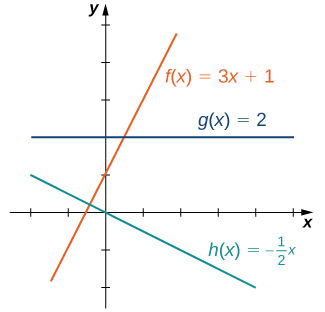Figure 1. These linear functions are increasing or decreasing on $(-\infty, \infty)$ and one function is a horizontal line.

As suggested by (Figure), the graph of any linear function is a line. One of the distinguishing features of a line is its slope. The slope is the change in $y$ for each unit change in $x$. The slope measures both the steepness and the direction of a line. If the slope is positive, the line points upward when moving from left to right. If the slope is negative, the line points downward when moving from left to right. If the slope is zero, the line is horizontal. To calculate the slope of a line, we need to determine the ratio of the change in $y$ versus the change in $x$. To do so, we choose any two points $(x_1,y_1)$ and $(x_2,y_2)$ on the line and calculate $\frac{y_2-y_1}{x_2-x_1}$. In (Figure), we see this ratio is independent of the points chosen.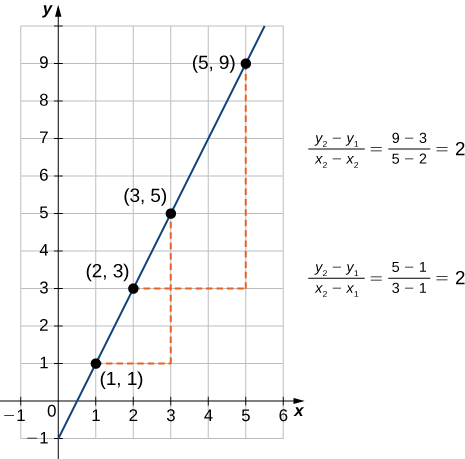Figure 2. or any linear function, the slope $(y_2-y_1)/(x_2-x_1)$ is independent of the choice of points $(x_1,y_1)$ and $(x_2,y_2)$ on the line.

### Definition

Consider line $L$ passing through points $(x_1,y_1)$ and $(x_2,y_2)$. Let $\Delta y=y_2-y_1$ and $\Delta x=x_2-x_1$ denote the changes in $y$ and $x$, respectively. The slope of the line is

$m=\frac{y_2-y_1}{x_2-x_1}=\frac{\Delta y}{\Delta x}$.

We now examine the relationship between slope and the formula for a linear function. Consider the linear function given by the formula $f(x)=ax+b$. As discussed earlier, we know the graph of a linear function is given by a line. We can use our definition of slope to calculate the slope of this line. As shown, we can determine the slope by calculating $(y_2-y_1)/(x_2-x_1)$ for any points $(x_1,y_1)$ and $(x_2,y_2)$ on the line. Evaluating the function $f$ at $x=0$, we see that $(0,b)$ is a point on this line. Evaluating this function at $x=1$, we see that $(1,a+b)$ is also a point on this line. Therefore, the slope of this line is

$\frac{(a+b)-b}{1-0}=a$.

We have shown that the coefficient $a$ is the slope of the line. We can conclude that the formula $f(x)=ax+b$ describes a line with slope $a$. Furthermore, because this line intersects the $y$-axis at the point $(0,b)$, we see that the $y$-intercept for this linear function is $(0,b)$. We conclude that the formula $f(x)=ax+b$ tells us the slope, $a$, and the $y$-intercept, $(0,b)$, for this line. Since we often use the symbol $m$ to denote the slope of a line, we can write

$f(x)=mx+b$

to denote the slope-intercept form of a linear function.

Sometimes it is convenient to express a linear function in different ways. For example, suppose the graph of a linear function passes through the point $(x_1,y_1)$ and the slope of the line is $m$. Since any other point $(x,f(x))$ on the graph of $f$ must satisfy the equation

$m=\frac{f(x)-y_1}{x-x_1}$,

this linear function can be expressed by writing

$f(x)-y_1=m(x-x_1)$.

We call this equation the point-slope equation for that linear function.

Since every nonvertical line is the graph of a linear function, the points on a nonvertical line can be described using the slope-intercept or point-slope equations. However, a vertical line does not represent the graph of a function and cannot be expressed in either of these forms. Instead, a vertical line is described by the equation $x=k$ for some constant $k$. Since neither the slope-intercept form nor the point-slope form allows for vertical lines, we use the notation

$ax+by=c$,

where $a,b$ are both not zero, to denote the standard form of a line.

### Definition

Consider a line passing through the point $(x_1,y_1)$ with slope $m$. The equation

$y-y_1=m(x-x_1)$

is the point-slope equation for that line.

Consider a line with slope $m$ and $y$-intercept $(0,b)$. The equation

$y=mx+b$

is an equation for that line in slope-intercept form.

The standard form of a line is given by the equation

$ax+by=c$,

where $a$ and $b$ are both not zero. This form is more general because it allows for a vertical line, $x=k$.

### Finding the Slope and Equations of Lines

Consider the line passing through the points $(11,-4)$ and $(-4,5)$, as shown in (Figure).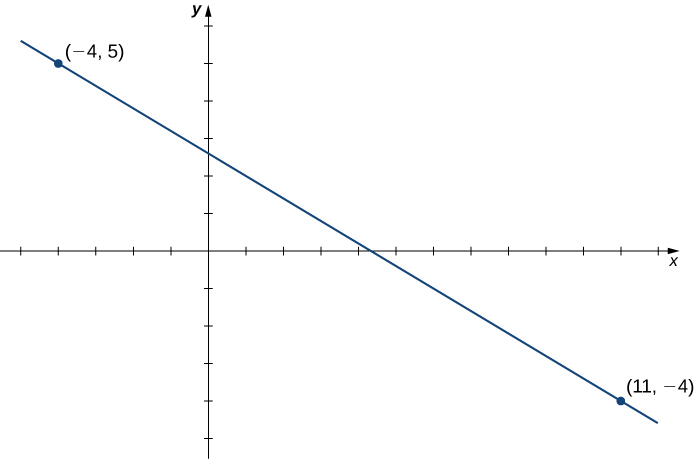Figure 3. Finding the equation of a linear function with a graph that is a line between two given points.

1. Find the slope of the line.
2. Find an equation for this linear function in point-slope form.
3. Find an equation for this linear function in slope-intercept form.

Consider the line passing through points $(-3,2)$ and $(1,4)$. Find the slope of the line.

Find an equation of that line in point-slope form. Find an equation of that line in slope-intercept form.

#### Hint

The slope $m=\Delta y / \Delta x$.

### A Linear Distance Function

Jessica leaves her house at 5:50 a.m. and goes for a 9-mile run. She returns to her house at 7:08 a.m. Answer the following questions, assuming Jessica runs at a constant pace.

1. Describe the distance $D$ (in miles) Jessica runs as a linear function of her run time $t$ (in minutes).
2. Sketch a graph of $D$.
3. Interpret the meaning of the slope.

# Polynomials

A linear function is a special type of a more general class of functions: polynomials. A polynomial function is any function that can be written in the form

$f(x)=a_nx^n+a_{n-1}x^{n-1}+\cdots+a_1x+a_0$

for some integer $n\ge 0$ and constants $a_n, \, a_{n-1}, \cdots, a_0$, where $a_n\ne 0$. In the case when $n=0$, we allow for $a_0=0$; if $a_0=0$, the function $f(x)=0$ is called the zero function. The value $n$ is called the degree of the polynomial; the constant $a_n$ is called the leading coefficient. A linear function of the form $f(x)=mx+b$ is a polynomial of degree 1 if $m\ne 0$ and degree 0 if $m=0$. A polynomial of degree 0 is also called a constant function. A polynomial function of degree 2 is called a quadratic function. In particular, a quadratic function has the form $f(x)=ax^2+bx+c$, where $a\ne 0$. A polynomial function of degree 3 is called a cubic function.

## Power Functions

Some polynomial functions are power functions. A power function is any function of the form $f(x)=ax^b$, where $a$ and $b$ are any real numbers. The exponent in a power function can be any real number, but here we consider the case when the exponent is a positive integer. (We consider other cases later.) If the exponent is a positive integer, then $f(x)=ax^n$ is a polynomial. If $n$ is even, then $f(x)=ax^n$ is an even function because $f(−x)=a(−x)^n=ax^n$ if $n$ is even. If $n$ is odd, then $f(x)=ax^n$ is an odd function because $f(−x)=a(−x)^n=−ax^n$ if $n$ is odd ((Figure)).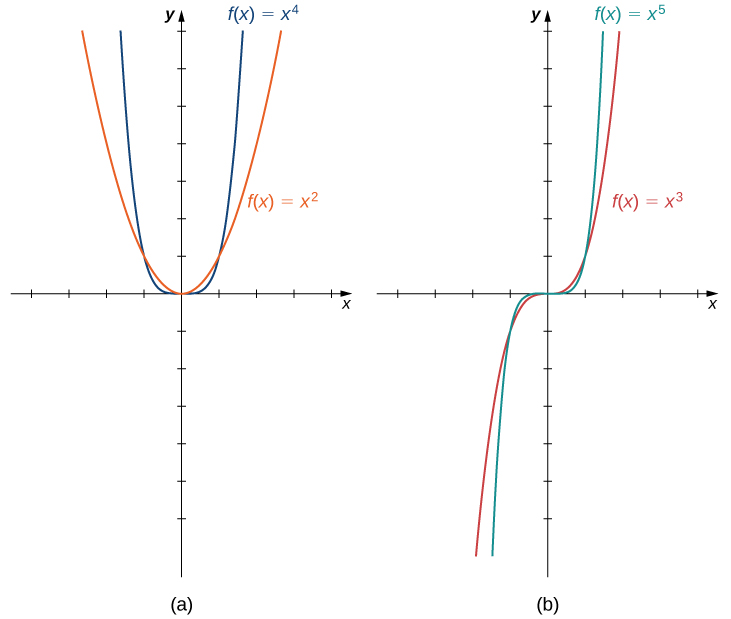Figure 4. (a) For any even integer $n,f(x)=ax^n$ is an even function. (b) For any odd integer $n,f(x)=ax^n$ is an odd function.

## Behavior at Infinity

To determine the behavior of a function $f$ as the inputs approach infinity, we look at the values $f(x)$ as the inputs, $x$, become larger. For some functions, the values of $f(x)$ approach a finite number. For example, for the function $f(x)=2+1/x$, the values $1/x$ become closer and closer to zero for all values of $x$ as they get larger and larger. For this function, we say “$f(x)$ approaches two as $x$ goes to infinity,” and we write $f(x)\to 2$ as $x\to \infty$. The line $y=2$ is a horizontal asymptote for the function $f(x)=2+1/x$ because the graph of the function gets closer to the line as $x$ gets larger.

For other functions, the values $f(x)$ may not approach a finite number but instead may become larger for all values of $x$ as they get larger. In that case, we say “$f(x)$ approaches infinity as $x$ approaches infinity,” and we write $f(x)\to \infty$ as $x\to \infty$. For example, for the function $f(x)=3x^2$, the outputs $f(x)$ become larger as the inputs $x$ get larger. We can conclude that the function $f(x)=3x^2$ approaches infinity as $x$ approaches infinity, and we write $3x^2\to \infty$ as $x\to \infty$. The behavior as $x \to −\infty$ and the meaning of $f(x) \to −\infty$ as $x \to \infty$ or $x \to −\infty$ can be defined similarly. We can describe what happens to the values of $f(x)$ as $x \to \infty$ and as $x \to −\infty$ as the end behavior of the function.

To understand the end behavior for polynomial functions, we can focus on quadratic and cubic functions. The behavior for higher-degree polynomials can be analyzed similarly. Consider a quadratic function $f(x)=ax^2+bx+c$. If $a>0$, the values $f(x) \to \infty$ as $x \to \pm \infty$. If $a<0$, the values $f(x) \to −\infty$ as $x \to \pm \infty$. Since the graph of a quadratic function is a parabola, the parabola opens upward if $a>0$; the parabola opens downward if $a<0$. (See (Figure)(a).)

Now consider a cubic function $f(x)=ax^3+bx^2+cx+d$. If $a>0$, then $f(x) \to \infty$ as $x \to \infty$ and $f(x) \to -\infty$ as $x \to −\infty$. If $a<0$, then $f(x) \to −\infty$ as $x \to \infty$ and $f(x) \to \infty$ as $x \to −\infty$. As we can see from both of these graphs, the leading term of the polynomial determines the end behavior. (See (Figure)(b).)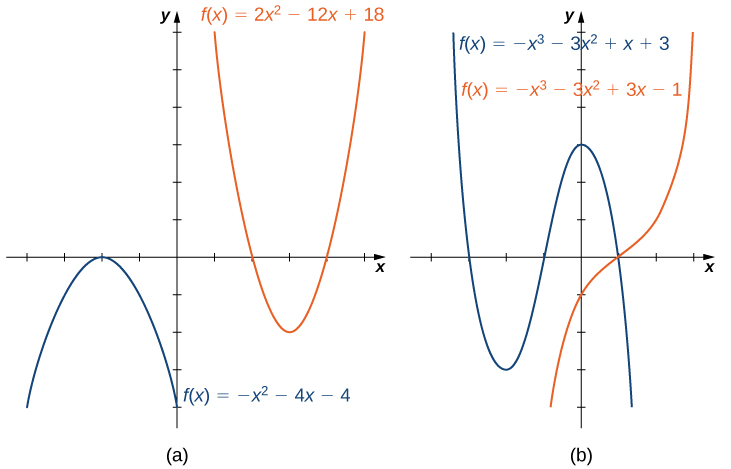Figure 5. (a) For a quadratic function, if the leading coefficient $a>0$, the parabola opens upward. If $a<0$, the parabola opens downward. (b) For a cubic function $f$, if the leading coefficient $a>0$, the values $f(x) \to \infty$ as $x\to \infty$ and the values $f(x)\to −\infty$ as $x \to −\infty$. If the leading coefficient $a<0$, the opposite is true.

## Zeros of Polynomial Functions

Another characteristic of the graph of a polynomial function is where it intersects the $x$-axis. To determine where a function $f$ intersects the $x$-axis, we need to solve the equation $f(x)=0$ for $x$. In the case of the linear function $f(x)=mx+b$, the $x$-intercept is given by solving the equation $mx+b=0$. In this case, we see that the $x$-intercept is given by $(−b/m,0)$. In the case of a quadratic function, finding the $x$-intercept(s) requires finding the zeros of a quadratic equation: $ax^2+bx+c=0$. In some cases, it is easy to factor the polynomial $ax^2+bx+c$ to find the zeros. If not, we make use of the quadratic formula.

### Rule: The Quadratic Formula

Consider the quadratic equation

$ax^2+bx+c=0$,

where $a\ne 0$. The solutions of this equation are given by the quadratic formula

$x=\frac{−b \pm \sqrt{b^2-4ac}}{2a}$.

If the discriminant $b^2-4ac>0$, this formula tells us there are two real numbers that satisfy the quadratic equation. If $b^2-4ac=0$, this formula tells us there is only one solution, and it is a real number. If $b^2-4ac<0$, no real numbers satisfy the quadratic equation.

In the case of higher-degree polynomials, it may be more complicated to determine where the graph intersects the $x$-axis. In some instances, it is possible to find the $x$-intercepts by factoring the polynomial to find its zeros. In other cases, it is impossible to calculate the exact values of the $x$-intercepts. However, as we see later in the text, in cases such as this, we can use analytical tools to approximate (to a very high degree) where the $x$-intercepts are located. Here we focus on the graphs of polynomials for which we can calculate their zeros explicitly.

### Graphing Polynomial Functions

For the following functions a. and b., i. describe the behavior of $f(x)$ as $x \to \pm \infty$, ii. find all zeros of $f$, and iii. sketch a graph of $f$.

1. $f(x)=-2x^2+4x-1$
2. $f(x)=x^3-3x^2-4x$

Consider the quadratic function $f(x)=3x^2-6x+2$. Find the zeros of $f$. Does the parabola open upward or downward?

#### Hint

Use the quadratic formula.

## Mathematical Models

A large variety of real-world situations can be described using mathematical models. A mathematical model is a method of simulating real-life situations with mathematical equations. Physicists, engineers, economists, and other researchers develop models by combining observation with quantitative data to develop equations, functions, graphs, and other mathematical tools to describe the behavior of various systems accurately. Models are useful because they help predict future outcomes. Examples of mathematical models include the study of population dynamics, investigations of weather patterns, and predictions of product sales.

As an example, let’s consider a mathematical model that a company could use to describe its revenue for the sale of a particular item. The amount of revenue $R$ a company receives for the sale of $n$ items sold at a price of $p$ dollars per item is described by the equation $R=p·n$. The company is interested in how the sales change as the price of the item changes. Suppose the data in (Figure) show the number of units a company sells as a function of the price per item.

 $\mathbf{p}$ 6 8 10 12 14 $\mathbf{n}$ 19.4 18.5 16.2 13.8 12.2

In (Figure), we see the graph the number of units sold (in thousands) as a function of price (in dollars). We note from the shape of the graph that the number of units sold is likely a linear function of price per item, and the data can be closely approximated by the linear function $n=-1.04p+26$ for $0\le p\le 25$, where $n$ predicts the number of units sold in thousands. Using this linear function, the revenue (in thousands of dollars) can be estimated by the quadratic function

$R(p)=p·(-1.04p+26)=-1.04p^2+26p$

for $0\le p\le 25$. In Example, we use this quadratic function to predict the amount of revenue the company receives depending on the price the company charges per item. Note that we cannot conclude definitively the actual number of units sold for values of $p$, for which no data are collected. However, given the other data values and the graph shown, it seems reasonable that the number of units sold (in thousands) if the price charged is $p$ dollars may be close to the values predicted by the linear function $n=-1.04p+26$.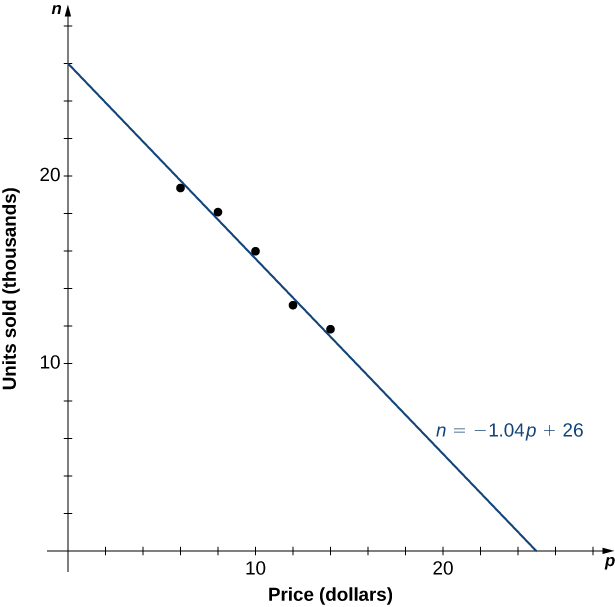Figure 6. The data collected for the number of items sold as a function of price is roughly linear. We use the linear function $n=-1.04p+26$ to estimate this function.

### Maximizing Revenue

A company is interested in predicting the amount of revenue it will receive depending on the price it charges for a particular item. Using the data from (Figure), the company arrives at the following quadratic function to model revenue $R$ as a function of price per item $p$:

$R(p)=p·(-1.04p+26)=-1.04p^2+26p$

for $0\le p\le 25$.

1. Predict the revenue if the company sells the item at a price of $p=\5$ and $p=\17$.
2. Find the zeros of this function and interpret the meaning of the zeros.
3. Sketch a graph of $R$.
4. Use the graph to determine the value of $p$ that maximizes revenue. Find the maximum revenue.

# Algebraic Functions

By allowing for quotients and fractional powers in polynomial functions, we create a larger class of functions. An algebraic function is one that involves addition, subtraction, multiplication, division, rational powers, and roots. Two types of algebraic functions are rational functions and root functions.

Just as rational numbers are quotients of integers, rational functions are quotients of polynomials. In particular, a rational function is any function of the form $f(x)=p(x)/q(x)$, where $p(x)$ and $q(x)$ are polynomials. For example,

$f(x)=\frac{3x-1}{5x+2}$ and $g(x)=\frac{4}{x^2+1}$

are rational functions. A root function is a power function of the form $f(x)=x^{1/n}$, where $n$ is a positive integer greater than one. For example, $f(x)=x^{1/2}=\sqrt{x}$ is the square-root function and $g(x)=x^{1/3}=\sqrt{x}$ is the cube-root function. By allowing for compositions of root functions and rational functions, we can create other algebraic functions. For example, $f(x)=\sqrt{4-x^2}$ is an algebraic function.

### Finding Domain and Range for Algebraic Functions

For each of the following functions, find the domain and range.

1. $f(x)=\frac{3x-1}{5x+2}$
2. $f(x)=\sqrt{4-x^2}$

Find the domain and range for the function $f(x)=(5x+2)/(2x-1)$.

#### Hint

The denominator cannot be zero. Solve the equation $y=(5x+2)/(2x-1)$ for $x$ to find the range.

The root functions $f(x)=x^{1/n}$ have defining characteristics depending on whether $n$ is odd or even. For all even integers $n \ge 2$, the domain of $f(x)=x^{1/n}$ is the interval $[0,\infty)$. For all odd integers $n \ge 1$, the domain of $f(x)=x^{1/n}$ is the set of all real numbers. Since $x^{1/n}=(−x)^{1/n}$ for odd integers $n, \, f(x)=x^{1/n}$ is an odd function if $n$ is odd. See the graphs of root functions for different values of $n$ in (Figure).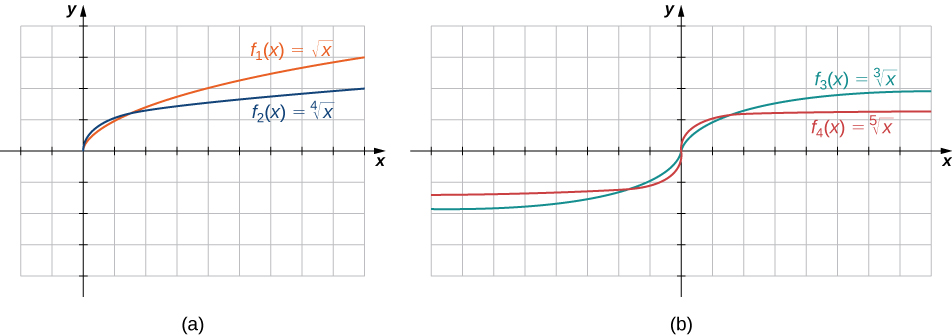Figure 7. (a) If $n$ is even, the domain of $f(x)=\sqrt[n]{x}$ is $[0,\infty)$. (b) If $n$ is odd, the domain of $f(x)=\sqrt[n]{x}$ is $(-\infty,\infty )$ and the function $f(x)=\sqrt[n]{x}$ is an odd function.

### Finding Domains for Algebraic Functions

For each of the following functions, determine the domain of the function.

1. $f(x)=\frac{3}{x^2-1}$
2. $f(x)=\frac{2x+5}{3x^2+4}$
3. $f(x)=\sqrt{4-3x}$
4. $f(x)=\sqrt{2x-1}$

Find the domain for each of the following functions: $f(x)=(5-2x)/(x^2+2)$ and $g(x)=\sqrt{5x-1}$.

#### Hint

Determine the values of $x$ when the expression in the denominator of $f$ is nonzero, and find the values of $x$ when the expression inside the radical of $g$ is nonnegative.

# Transcendental Functions

Thus far, we have discussed algebraic functions. Some functions, however, cannot be described by basic algebraic operations. These functions are known as transcendental functions because they are said to “transcend,” or go beyond, algebra. The most common transcendental functions are trigonometric, exponential, and logarithmic functions. A trigonometric function relates the ratios of two sides of a right triangle. They are $\sin x,\, \cos x, \, \tan x, \, \cot x,\, \sec x$, and $\csc x$. (We discuss trigonometric functions later in the chapter.) An exponential function is a function of the form $f(x)=b^x$, where the base $b>0, \, b \ne 1$. A logarithmic function is a function of the form $f(x)=\log_b(x)$ for some constant $b>0, \, b \ne 1$, where $\log_b(x)=y$ if and only if $b^y=x$. (We also discuss exponential and logarithmic functions later in the chapter.)

### Classifying Algebraic and Transcendental Functions

Classify each of the following functions, a. through c., as algebraic or transcendental.

1. $f(x)= \large \frac{\sqrt{x^3+1}}{4x+2}$
2. $f(x)=2^{x^2}$
3. $f(x)=\sin (2x)$

Is $f(x)=x/2$ an algebraic or a transcendental function?

# Piecewise-Defined Functions

Sometimes a function is defined by different formulas on different parts of its domain. A function with this property is known as a piecewise-defined function. The absolute value function is an example of a piecewise-defined function because the formula changes with the sign of $x$:

$f(x)=\begin{cases} x, & x \ge 0 \\ -x, & x < 0 \end{cases}$.

Other piecewise-defined functions may be represented by completely different formulas, depending on the part of the domain in which a point falls. To graph a piecewise-defined function, we graph each part of the function in its respective domain, on the same coordinate system. If the formula for a function is different for $x<a$ and $x>a$, we need to pay special attention to what happens at $x=a$ when we graph the function. Sometimes the graph needs to include an open or closed circle to indicate the value of the function at $x=a$. We examine this in the next example.

### Graphing a Piecewise-Defined Function

Sketch a graph of the following piecewise-defined function:

$f(x)=\begin{cases} x+3, & x < 1 \\ (x-2)^2 & x \ge 1 \end{cases}$

Sketch a graph of the function

$f(x)=\begin{cases} 2-x, & x \le 2 \\ x+2, & x>2 \end{cases}$

#### Hint

Graph one linear function for $x \le 2$ and then graph a different linear function for $x>2$.

### Parking Fees Described by a Piecewise-Defined Function

In a big city, drivers are charged variable rates for parking in a parking garage. They are charged $10 for the first hour or any part of the first hour and an additional$2 for each hour or part thereof up to a maximum of $30 for the day. The parking garage is open from 6 a.m. to 12 midnight. 1. Write a piecewise-defined function that describes the cost $C$ to park in the parking garage as a function of hours parked $x$. 2. Sketch a graph of this function $C(x)$. The cost of mailing a letter is a function of the weight of the letter. Suppose the cost of mailing a letter is $49\text{¢}$ for the first ounce and $21\text{¢}$ for each additional ounce. Write a piecewise-defined function describing the cost $C$ as a function of the weight $x$ for $0 < x \le 3$, where $C$ is measured in cents and $x$ is measured in ounces. #### Hint The piecewise-defined function is constant on the intervals $(0,1], \, (1,2], \, \cdots$ # Transformations of Functions We have seen several cases in which we have added, subtracted, or multiplied constants to form variations of simple functions. In the previous example, for instance, we subtracted 2 from the argument of the function $y=x^2$ to get the function $f(x)=(x-2)^2$. This subtraction represents a shift of the function $y=x^2$ two units to the right. A shift, horizontally or vertically, is a type of transformation of a function. Other transformations include horizontal and vertical scalings, and reflections about the axes. A vertical shift of a function occurs if we add or subtract the same constant to each output $y$. For $c>0$, the graph of $f(x)+c$ is a shift of the graph of $f(x)$ up $c$ units, whereas the graph of $f(x)-c$ is a shift of the graph of $f(x)$ down $c$ units. For example, the graph of the function $f(x)=x^3+4$ is the graph of $y=x^3$ shifted up 4 units; the graph of the function $f(x)=x^3-4$ is the graph of $y=x^3$ shifted down 4 units ((Figure)).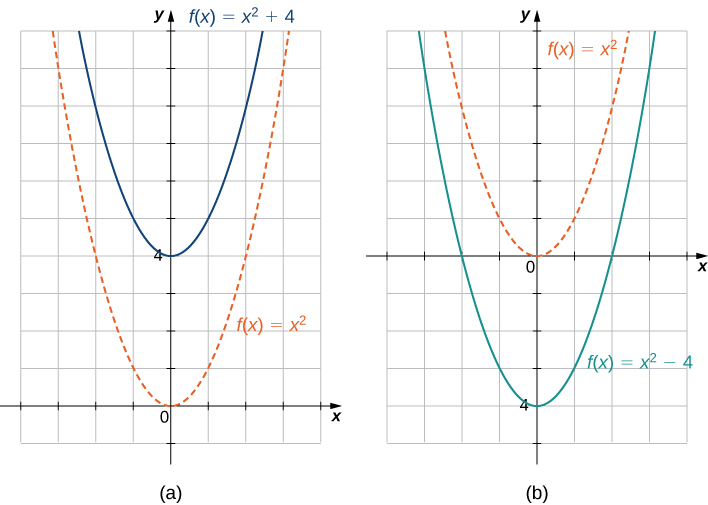Figure 8. (a) For $c>0$, the graph of $y=f(x)+c$ is a vertical shift up $c$ units of the graph of $y=f(x)$. (b) For $c>0$, the graph of $y=f(x)-c$ is a vertical shift down $c$ units of the graph of $y=f(x)$. A horizontal shift of a function occurs if we add or subtract the same constant to each input $x$. For $c>0$, the graph of $f(x+c)$ is a shift of the graph of $f(x)$ to the left $c$ units; the graph of $f(x-c)$ is a shift of the graph of $f(x)$ to the right $c$ units. Why does the graph shift left when adding a constant and shift right when subtracting a constant? To answer this question, let’s look at an example. Consider the function $f(x)=|x+3|$ and evaluate this function at $x-3.$ Since $f(x-3)=|x|$ and $x-3<x$, the graph of $f(x)=|x+3|$ is the graph of $y=|x|$ shifted left 3 units. Similarly, the graph of $f(x)=|x-3|$ is the graph of $y=|x|$ shifted right 3 units ((Figure)).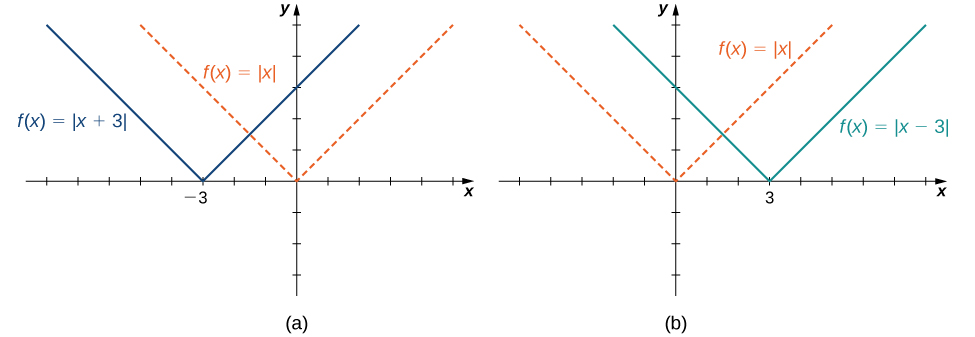Figure 9. (a) For $c>0$, the graph of $y=f(x+c)$ is a horizontal shift left $c$ units of the graph of $y=f(x)$. (b) For $c>0$, the graph of $y=f(x-c)$ is a horizontal shift right $c$ units of the graph of $y=f(x)$. A vertical scaling of a graph occurs if we multiply all outputs $y$ of a function by the same positive constant. For $c>0$, the graph of the function $cf(x)$ is the graph of $f(x)$ scaled vertically by a factor of $c$. If $c>1$, the values of the outputs for the function $cf(x)$ are larger than the values of the outputs for the function $f(x)$; therefore, the graph has been stretched vertically. If $0<c<1$, then the outputs of the function $cf(x)$ are smaller, so the graph has been compressed. For example, the graph of the function $f(x)=3x^2$ is the graph of $y=x^2$ stretched vertically by a factor of 3, whereas the graph of $f(x)=x^2/3$ is the graph of $y=x^2$ compressed vertically by a factor of 3 ((Figure)).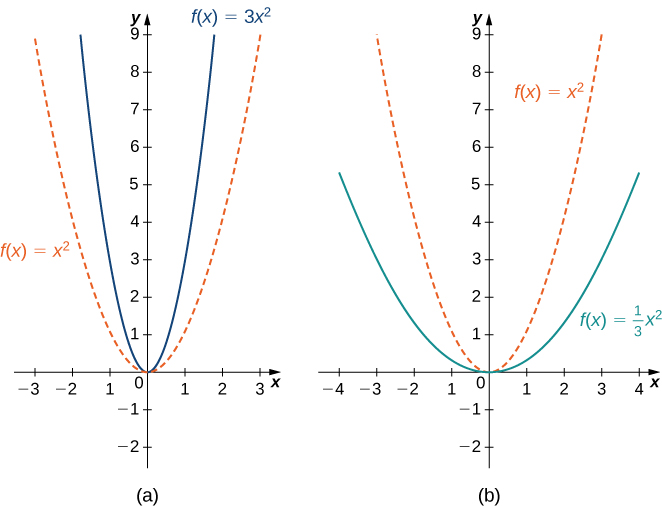Figure 10. (a) If $c>1$, the graph of $y=cf(x)$ is a vertical stretch of the graph of $y=f(x)$. (b) If $0<c<1$, the graph of $y=cf(x)$ is a vertical compression of the graph of $y=f(x)$. The horizontal scaling of a function occurs if we multiply the inputs $x$ by the same positive constant. For $c>0$, the graph of the function $f(cx)$ is the graph of $f(x)$ scaled horizontally by a factor of $c$. If $c>1$, the graph of $f(cx)$ is the graph of $f(x)$ compressed horizontally. If $0<c<1$, the graph of $f(cx)$ is the graph of $f(x)$ stretched horizontally. For example, consider the function $f(x)=\sqrt{2x}$ and evaluate $f$ at $x/2.$ Since $f(x/2)=\sqrt{x}$, the graph of $f(x)=\sqrt{2x}$ is the graph of $y=\sqrt{x}$ compressed horizontally. The graph of $y=\sqrt{x/2}$ is a horizontal stretch of the graph of $y=\sqrt{x}$ ((Figure)).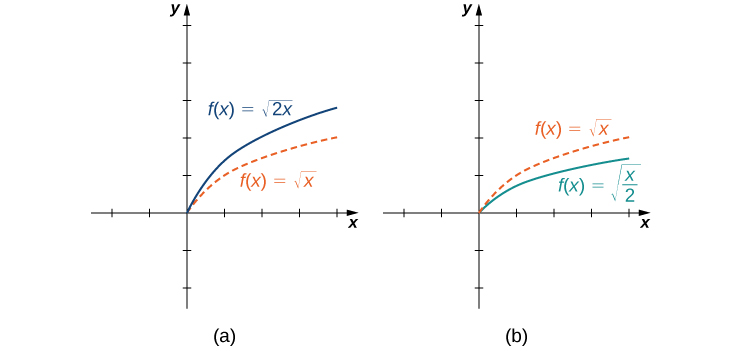Figure 11. (a) If $c>1$, the graph of $y=f(cx)$ is a horizontal compression of the graph of $y=f(x)$. (b) If $0<c<1$, the graph of $y=f(cx)$ is a horizontal stretch of the graph of $y=f(x)$. We have explored what happens to the graph of a function $f$ when we multiply $f$ by a constant $c>0$ to get a new function $cf(x)$. We have also discussed what happens to the graph of a function $f$ when we multiply the independent variable $x$ by $c>0$ to get a new function $f(cx)$. However, we have not addressed what happens to the graph of the function if the constant $c$ is negative. If we have a constant $c<0$, we can write $c$ as a positive number multiplied by -1; but, what kind of transformation do we get when we multiply the function or its argument by -1? When we multiply all the outputs by -1, we get a reflection about the $x$-axis. When we multiply all inputs by -1, we get a reflection about the $y$-axis. For example, the graph of $f(x)=−(x^3+1)$ is the graph of $y=(x^3+1)$ reflected about the $x$-axis. The graph of $f(x)=(−x)^3+1$ is the graph of $y=x^3+1$ reflected about the $y$-axis ((Figure)).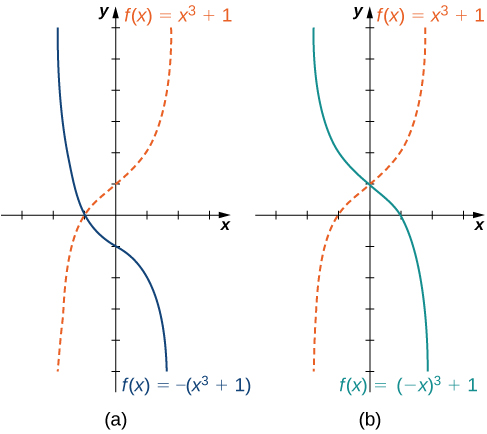Figure 12. (a) The graph of $y=−f(x)$ is the graph of $y=f(x)$ reflected about the $x$-axis. (b) The graph of $y=f(−x)$ is the graph of $y=f(x)$ reflected about the $y$-axis. If the graph of a function consists of more than one transformation of another graph, it is important to transform the graph in the correct order. Given a function $f(x)$, the graph of the related function $y=cf(a(x+b))+d$ can be obtained from the graph of $y=f(x)$ by performing the transformations in the following order. 1. Horizontal shift of the graph of $y=f(x)$. If $b>0$, shift left. If $b<0$, shift right. 2. Horizontal scaling of the graph of $y=f(x+b)$ by a factor of $|a|$. If $a<0$, reflect the graph about the $y$-axis. 3. Vertical scaling of the graph of $y=f(a(x+b))$ by a factor of $|c|$. If $c<0$, reflect the graph about the $x$-axis. 4. Vertical shift of the graph of $y=cf(a(x+b))$. If $d>0$, shift up. If $d<0$, shift down. We can summarize the different transformations and their related effects on the graph of a function in the following table. Transformations of Functions Transformation of $f(c>0)$ Effect on the graph of$f$ $f(x)+c$ Vertical shift up $c$ units $f(x)-c$ Vertical shift down $c$ units $f(x+c)$ Shift left by $c$ units $f(x-c)$ Shift right by $c$ units $cf(x)$ Vertical stretch if $c>1$; vertical compression if $0<c<1$ $f(cx)$ Horizontal stretch if $0<c<1$; horizontal compression if $c>1$ $−f(x)$ Reflection about the $x$-axis $f(−x)$ Reflection about the $y$-axis ### Transforming a Function For each of the following functions, a. and b., sketch a graph by using a sequence of transformations of a well-known function. 1. $f(x)=−|x+2|-3$ 2. $f(x)=3\sqrt{−x}+1$ Describe how the function $f(x)=−(x+1)^2-4$ can be graphed using the graph of $y=x^2$ and a sequence of transformations. #### Hint Use (Table). ### Key Concepts • The power function $f(x)=x^n$ is an even function if $n$ is even and $n \ne 0$, and it is an odd function if $n$ is odd. • The root function $f(x)=x^{1/n}$ has the domain $[0,\infty )$ if $n$ is even and the domain $(-\infty,\infty )$ if $n$ is odd. If $n$ is odd, then $f(x)=x^{1/n}$ is an odd function. • The domain of the rational function $f(x)=p(x)/q(x)$, where $p(x)$ and $q(x)$ are polynomial functions, is the set of $x$ such that $q(x) \ne 0$. • Functions that involve the basic operations of addition, subtraction, multiplication, division, and powers are algebraic functions. All other functions are transcendental. Trigonometric, exponential, and logarithmic functions are examples of transcendental functions. • A polynomial function $f$ with degree $n \ge 1$ satisfies $f(x) \to \pm \infty$ as $x \to \pm \infty$. The sign of the output as $x \to \infty$ depends on the sign of the leading coefficient only and on whether $n$ is even or odd. • Vertical and horizontal shifts, vertical and horizontal scalings, and reflections about the $x$– and $y$-axes are examples of transformations of functions. # Key Equations • Point-slope equation of a line $y-y_1=m(x-x_1)$ • Slope-intercept form of a line $y=mx+b$ • Standard form of a line $ax+by=c$ • Polynomial function $f(x)=a_nx^n+a_{n-1}x^{n-1}+ \cdots +a_1x+a_0$ For the following exercises, for each pair of points, a. find the slope of the line passing through the points and b. indicate whether the line is increasing, decreasing, horizontal, or vertical. 1. $(-2,4)$ and $(1,1)$ 2. $(-1,4)$ and $(3,-1)$ 3. $(3,5)$ and $(-1,2)$ 4. $(6,4)$ and $(4,-3)$ 5. $(2,3)$ and $(5,7)$ 6. $(1,9)$ and $(-8,5)$ 7. $(2,4)$ and $(1,4)$ 8. $(1,4)$ and $(1,0)$ For the following exercises, write the equation of the line satisfying the given conditions in slope-intercept form. 9. Slope $=-6$, passes through $(1,3)$ 10. Slope $=3$, passes through $(-3,2)$ 11. Slope $=\frac{1}{3}$, passes through $(0,4)$ 12. Slope $=\frac{2}{5}$, $x$-intercept $=8$ 13. Passing through $(2,1)$ and $(-2,-1)$ 14. Passing through $(-3,7)$ and $(1,2)$ 15. $x$-intercept $=5$ and $y$-intercept $=-3$ 16. $x$-Intercept $=-6$ and $y$-intercept $=9$ For the following exercises, for each linear equation, a. give the slope $m$ and $y$-intercept $b$, if any, and b. graph the line. 17. $y=2x-3$ 18. $y=-\frac{1}{7}x+1$ 19. $f(x)=-6x$ 20. $f(x)=-5x+4$ 21. $4y+24=0$ 22. $8x-4=0$ 23. $2x+3y=6$ 24. $6x-5y+15=0$ For the following exercises, for each polynomial, a. find the degree; b. find the zeros, if any; c. find the $y$-intercept(s), if any; d. use the leading coefficient to determine the graph’s end behavior; and e. determine algebraically whether the polynomial is even, odd, or neither. 25. $f(x)=2x^2-3x-5$ 26. $f(x)=-3x^2+6x$ 27. $f(x)=\frac{1}{2}x^2-1$ 28. $f(x)=x^3+3x^2-x-3$ 29. $f(x)=3x-x^3$ For the following exercises, use the graph of $f(x)=x^2$ to graph each transformed function $g$. 30. $g(x)=x^2-1$ 31. $g(x)=(x+3)^2+1$ For the following exercises, use the graph of $f(x)=\sqrt{x}$ to graph each transformed function $g$. 32. $g(x)=\sqrt{x+2}$ 33. $g(x)=−\sqrt{x}-1$ For the following exercises, use the graph of $y=f(x)$ to graph each transformed function $g$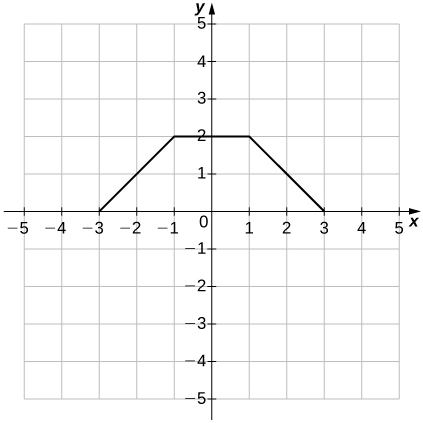34. $g(x)=f(x)+1$ 35. $g(x)=f(x-1)+2$ For the following exercises, for each of the piecewise-defined functions, a. evaluate at the given values of the independent variable and b. sketch the graph. 36. $f(x)=\begin{cases} 4x+3, & x \le 0 \\ -x+1, & x > 0 \end{cases}$; $f(-3); \, f(0); \, f(2)$ 37. $f(x)=\begin{cases}x^2-3, & x < 0 \\ 4x-3, & x \ge 0 \end{cases}$; $f(-4); \, f(0); \, f(2)$ 38. $h(x)=\begin{cases} x+1, & x \le 5 \\ 4, & x > 5 \end{cases}$; $h(0); \, h(\pi ); \, h(5)$ 39. $g(x)=\begin{cases} \large{\frac{3}{x-2}}, & x \ne 2 \\ 4, & x = 2 \end{cases}$; $g(0); \, g(-4); \, g(2)$ For the following exercises, determine whether the statement is true or false. Explain why. 40. $f(x)=(4x+1)/(7x-2)$ is a transcendental function. 41. $g(x)=\sqrt{x}$ is an odd root function 42. A logarithmic function is an algebraic function. 43. A function of the form $f(x)=x^b$, where $b$ is a real valued constant, is an exponential function. 44. The domain of an even root function is all real numbers. 45. [T] A company purchases some computer equipment for$20,500. At the end of a 3-year period, the value of the equipment has decreased linearly to $12,300. 1. Find a function $y=V(t)$ that determines the value $V$ of the equipment at the end of $t$ years. 2. Find and interpret the meaning of the $x$– and $y$-intercepts for this situation. 3. What is the value of the equipment at the end of 5 years? 4. When will the value of the equipment be$3000?

46. [T] Total online shopping during the Christmas holidays has increased dramatically during the past 5 years. In 2012 $(t=0)$, total online holiday sales were $42.3 billion, whereas in 2013 they were$48.1 billion.

1. Find a linear function $S$ that estimates the total online holiday sales in the year $t$.
2. Interpret the slope of the graph of $S$.
3. Use part a. to predict the year when online shopping during Christmas will reach $60 billion. 47. [T] A family bakery makes cupcakes and sells them at local outdoor festivals. For a music festival, there is a fixed cost of$125 to set up a cupcake stand. The owner estimates that it costs $0.75 to make each cupcake. The owner is interested in determining the total cost $C$ as a function of number of cupcakes made. 1. Find a linear function that relates cost $C$ to $x$, the number of cupcakes made. 2. Find the cost to bake 160 cupcakes. 3. If the owner sells the cupcakes for$1.50 apiece, how many cupcakes does she need to sell to start making profit? (Hint: Use the INTERSECTION function on a calculator to find this number.)

48. [T] A house purchased for $250,000 is expected to be worth twice its purchase price in 18 years. 1. Find a linear function that models the price $P$ of the house versus the number of years $t$ since the original purchase. 2. Interpret the slope of the graph of $P$. 3. Find the price of the house 15 years from when it was originally purchased. 49. [T] A car was purchased for$26,000. The value of the car depreciates by $1500 per year. 1. Find a linear function that models the value $V$ of the car after $t$ years. 2. Find and interpret $V(4)$. 50. [T] A condominium in an upscale part of the city was purchased for$432,000. In 35 years it is worth \$60,500. Find the rate of depreciation.

51. [T] The total cost $C$ (in thousands of dollars) to produce a certain item is modeled by the function $C(x)=10.50x+28,500$, where $x$ is the number of items produced. Determine the cost to produce 175 items.

52. [T] A professor asks her class to report the amount of time $t$ they spent writing two assignments. Most students report that it takes them about 45 minutes to type a four-page assignment and about 1.5 hours to type a nine-page assignment.

1. Find the linear function $y=N(t)$ that models this situation, where $N$ is the number of pages typed and $t$ is the time in minutes.
2. Use part a. to determine how many pages can be typed in 2 hours.
3. Use part a. to determine how long it takes to type a 20-page assignment.

53. [T] The output (as a percent of total capacity) of nuclear power plants in the United States can be modeled by the function $P(t)=1.8576t+68.052$, where $t$ is time in years and $t=0$ corresponds to the beginning of 2000. Use the model to predict the percentage output in 2015.

54. [T] The admissions office at a public university estimates that 65% of the students offered admission to the class of 2019 will actually enroll.

1. Find the linear function $y=N(x)$, where $N$ is the number of students that actually enroll and $x$ is the number of all students offered admission to the class of 2019.
2. If the university wants the 2019 freshman class size to be 1350, determine how many students should be admitted.

## Glossary

algebraic function
a function involving any combination of only the basic operations of addition, subtraction, multiplication, division, powers, and roots applied to an input variable $x$
cubic function
a polynomial of degree 3; that is, a function of the form $f(x)=ax^3+bx^2+cx+d$, where $a \ne 0$
degree
for a polynomial function, the value of the largest exponent of any term
linear function
a function that can be written in the form $f(x)=mx+b$
logarithmic function
a function of the form $f(x)=\log_b(x)$ for some base $b>0, \, b \ne 1$ such that $y=\log_b(x)$ if and only if $b^y=x$
mathematical model
A method of simulating real-life situations with mathematical equations
piecewise-defined function
a function that is defined differently on different parts of its domain
point-slope equation
equation of a linear function indicating its slope and a point on the graph of the function
polynomial function
a function of the form $f(x)=a_nx^n+a_{n-1}x^{n-1}+ \cdots +a_1x+a_0$
power function
a function of the form $f(x)=x^n$ for any positive integer $n \ge 1$
quadratic function
a polynomial of degree 2; that is, a function of the form $f(x)=ax^2+bx+c$ where $a \ne 0$
rational function
a function of the form $f(x)=p(x)/q(x)$, where $p(x)$ and $q(x)$ are polynomials
root function
a function of the form $f(x)=x^{1/n}$ for any integer $n \ge 2$
slope
the change in $y$ for each unit change in $x$
slope-intercept form
equation of a linear function indicating its slope and $y$-intercept
transcendental function
a function that cannot be expressed by a combination of basic arithmetic operations
transformation of a function
a shift, scaling, or reflection of a function